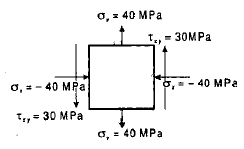Courses

# Test: Strength Of Material (Level- 1)- 1

## 25 Questions MCQ Test Civil Engineering SSC JE (Technical) | Test: Strength Of Material (Level- 1)- 1

Description
This mock test of Test: Strength Of Material (Level- 1)- 1 for Civil Engineering (CE) helps you for every Civil Engineering (CE) entrance exam. This contains 25 Multiple Choice Questions for Civil Engineering (CE) Test: Strength Of Material (Level- 1)- 1 (mcq) to study with solutions a complete question bank. The solved questions answers in this Test: Strength Of Material (Level- 1)- 1 quiz give you a good mix of easy questions and tough questions. Civil Engineering (CE) students definitely take this Test: Strength Of Material (Level- 1)- 1 exercise for a better result in the exam. You can find other Test: Strength Of Material (Level- 1)- 1 extra questions, long questions & short questions for Civil Engineering (CE) on EduRev as well by searching above.
QUESTION: 1

Solution:
QUESTION: 2

Solution:
QUESTION: 3

### Hook's law holds good up to

Solution:

Hooke's law states that : The Force applied to an elastic material is directly proportional to the extension produced.

We can write this as :

F = ke

Where k is the spring constant, F the force and e the extension.

For Hooke's law to hold the extension and Force must always proportional.

If the proportionality is broken then Hooke's law becomes invalid.

Therefore Hooke's law holds good up to limit of proportionality.

QUESTION: 4

The deformation of a bar under its own weight is __________ the deformation, if the same body is subjected to a direct load equal to weight of the body.

Solution:
QUESTION: 5

The elongation of a conical bar under its own weight is ......that of prismatic bar of the same length.

Solution:

Correct Answer :- c

Explanation : Conical bar/Prismatic Bar.

= (wl2/6E) / (wl2/2E),

=1/3.

QUESTION: 6

Modular ratio of the two materials is the ratio of

Solution:
QUESTION: 7

A bolt is made to pass through a tube and both of them are tightly fitted with the holp of washers and nuts. If the nut is tightened, then

Solution:
QUESTION: 8

The Poisson's ratio for cast iron varies from

Solution:
QUESTION: 9

The Poisson's ratio for stainless steel varies from

Solution:
QUESTION: 10

When a body is subjected to three mutually perpendicular stresses, of equal intensity, the ratio of direct stress to the corresponding volumetric strain is known as

Solution:
QUESTION: 11

The relation between Young's modulus (E) and bulk modulus (K) is given by

Solution:
QUESTION: 12

The ratio of shear modulus to the modulus of elasticity for a Poisson's ratio of 0.4 will be

Solution:
QUESTION: 13

The relation between Young's modulus (E), shear modulus (C) and bulk modulus (K) is given by

Solution:
QUESTION: 14

When a body is subjected to a direct tensile stress (sx) in one plane accompanied by a simple
shear stress (txy), the maximum shear stress is

Solution:
QUESTION: 15

The state of stress at a point in a loaded member is shown is fig. The magnitude of maximum shear stress isSolution:
QUESTION: 16

The strain energy stored in a spring, when subjected to maximum load, without suffering permanent distortion, is known as

Solution:
QUESTION: 17

The bending equation is

Solution:
QUESTION: 18

The neutral axis of the cross-section a beam is that axis at which the bending stress is

Solution:
QUESTION: 19

When a rectangular beam is loaded transversely, the maximum compressive stress is developed on the

Solution:
QUESTION: 20

Two closely coiled helical springs 'A' and 'B' are equal in all respects but the diameter of wire of spring 'A' is double that of spring 'B' The stiffness of spring 'B' will be __________ that of spring 'A'

Solution:
QUESTION: 21

Two closely -coiled helical springs 'A' and 'B' are equal in all respects but the number of turns of spring 'A' is double that of spring 'B'. The stiffness of spring 'A' will be ....... that of spring 'B'.

Solution:

Stiffness is inversely proportional to the no. of turns

Therefore,option d will be the correct answer.

QUESTION: 22

A closely-coiled helical spring is cut into two halves. The stiffness of the resulting spring will be

Solution:
QUESTION: 23

A composite shaft consisting of two stepped portions having spring constants k1 and k2 is held between two rigid supports at the ends. Its equivalent spring constant is

Solution:
QUESTION: 24

A composite shaft consisting of two stepped portions having spring constants k1 and k2 is held between two rigid supports at the ends. Its equivalent spring constant is

Solution:
QUESTION: 25

The maximum deflection of a cantilever beam of length l with a uniformly distributed load of w per unit length is (where W = wl)

Solution: